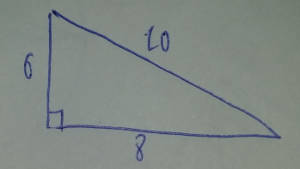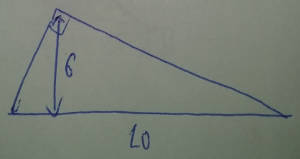## Yan Han's blog

On Computer Technology

 Tags

A while back, this answer by Job Bouwman appeared in my Quora digest and I enjoyed reading it (Thank you Job!) so much that I intended to blog about some of the more tricky parts of the answer where I thought some explanations got skipped over.

The question is:

A right triangle has a hypotenuse equal to 10 and an altitude to the hypotenuse equal to 6. Find the area of the triangle.

Like many other people, I thought it was $$10 * 6 * \frac{1}{2} = 30$$ but was quite shocked when it turned out wrong. It is due to a misinterpretation of the following:

altitude to the hypotenuse equal to 6

I interpreted this as the height of the triangle. In otherwords, a triangle like this:Let’s clarify what altitude to the hypotenuse means.

Based on the Wikpedia entry on the Altitude of a triangle:

In geometry, an altitude of a triangle is the line segment through a vertex and perpendicular to (ie. forming a right angle with) a line containing the base (the opposite side of the triangle).

Therefore, the triangle should look something like this:But we are told that such a triangle cannot exist. Why? By looking at:We see that the hypotenuse of all the right angle triangles is $$10$$, which also happens to be the diameter of the circle. There are 2 edges other than the hypotenuse and both edges start at the opposite ends of the hypotenuse and meet at a point on the circle. At this point I wondered: Hmm, must triangles like these, ie triangles where one edge is the diameter of a circle, and the other 2 edges start from opposite ends of the hypotenuse and meet at the radius, always be right angle triangles?

A google search on “triangle with hypotenuse being diameter of circle always right angle” yields the following Wikipedia page on Thale’s theorem as the first result, which is exactly what we hoped is true (Google is just amazing). I highly recommend you read the proofs of Thales theorem on the Wiki page - it’s a pretty insightful read, although I can’t see how to extend the second proof to non unit circles.

Now, this means that any right angle triangle with hypotenuse $$10$$ has a maximum altitude to the hypotenuse of $$5$$, since $$5$$ is the radius of the circle and is the absolute maximum the altitude to the hypotenuse can go with the triangle still within the circle. In a very hand-wavy fashion, we can see that if we try to increase the altitude of the hypotenuse so the triangle juts out of the circle, the triangle will no longer be right angled. Similarly, if we increase the altitude of the hypotenuse such that it juts out of the circle but we insist on having it being right angled, then the hypotenuse of the triangle will exceed $$10$$. I do not have the math chops or the will to prove this so hopefully this is a reasonable explanation.

With that, the situation seems hopeless. A right angle triangle with hypotenuse $$10$$ simply cannot have an altitude to the hypotenuse greater than $$5$$.

However, Mathematics and human ingenuity often surprises us. This is no exception. So far we’ve only been considering Euclidean geometry. Let’s step into the 3D realm (this is the hard part of coming up with the answer; I also cannot explain how people come up with these insights).

Now, consider this (ignore the $$radius = \frac{20}{\pi}$$ part for now):Imagine you have a deflated balloon. Draw a triangle on it. Now inflate the balloon to a sphere. The edges of the triangle you drew on the balloon will look curved and the triangle will not look like a triangle on a 2D plane, but it is still a triangle. Deflate the balloon and you get back the same triangle - recognize that whether the balloon is deflated or inflated, the triangle did not change - it just looks different to your eyes when the balloon is deflated vs. when it is inflated. The moral of the story is this: if we take any 2 points on the surface of a sphere and connect them with a straight line on the surface, that straight line will looked curved.

But it seems that we can draw some triangles on the surface of the sphere that will not be triangles on the 2D plane when we deflate the sphere. Hence it is important to realize this: as long as we have a polygon that has 3 vertices and any of the 2 vertices are connected by straight lines on the sphere, we have a triangle on the sphere. This triangle may look strange compared to one on a 2D plane but it is a triangle.

Let $$A$$, $$B$$ be on the equator and $$C$$ be on the north pole. Let $$AC$$ be the straight line on the surface of the sphere joining points $$A$$ and $$C$$. Let $$BC$$ be the straight line on the surface of the sphere joining points $$B$$ and $$C$$. Let $$AB$$ be the straight line on the surface of the sphere joining points $$A$$ and $$B$$. We have ourselves a triangle in $$ABC$$.

The circle formed by extending the arc $$AC$$ is perpendicular to the circle formed by extending the arc $$AB$$, so $$\angle CAB$$ is a right angle and hence the triangle $$ABC$$ is a right angle triangle. Similarly, the circle formed by extending the arc $$BC$$ is perpendicular to the circle formed by extending the arc $$AB$$ so angle $$\angle CBA$$ is also a right angle. Let $$AC$$ be the hypotenuse of length $$10$$. Since $$BC$$ has one end on the north pole and the other on the equator, it also has length $$10$$. $$AB$$ is a valid altitude to the hypotenuse $$AC$$, since point $$B$$ is a vertex on the triangle and point $$A$$ sits at the hypotenuse and $$AB$$ is perpendicular to the hypotenuse $$AC$$. So there we go: we have a right angle triangle whose hypotenuse is $$10$$ and and altitude to the hypotenuse is $$6$$.

To compute the area of this triangle, the key lies in computing its radius. $$C$$ is the north pole while $$A$$ lies on the equator, so this arc is $$\frac{1}{4}$$ of the great circle formed by extending $$AC$$. Hence the great circle has circumference $$40$$, which is given by $$\pi * d$$, where $$d$$ is the diameter of the great circle and hence the sphere. Hence $$d = \frac{40}{\pi}$$ and the radius $$r = \frac{1}{2} * d = \frac{1}{2} * \frac{40}{\pi} = \frac{20}{\pi}$$, which is the number shown in the image above.

We see that our triangle perfectly covers a portion on the upper hemisphere. To be exact, since the diameter of any great circle of the sphere is $$40$$, our triangle covers $$\frac{6}{40}$$ of the area of the upper hemisphere. The surface area of the sphere is given by $$4 * \pi * r^{2}$$, and the upper hemisphere has area half of that, which is $$2 * \pi * r^{2}$$ and our triangle has area $$\frac{6}{40}$$ of that, which is $$\frac{6}{40} * 2 * \pi * (\frac{20}{\pi})^{2} = \frac{120}{\pi}$$. Ingenious.

## Credits

Big thanks to by Job Bouwman for his answer. The relevant images on Quora belongs to Quora and Job Bouwman.

Disclaimer: Opinions expressed on this blog are solely my own and do not express the views or opinions of my employer(s), past or present.